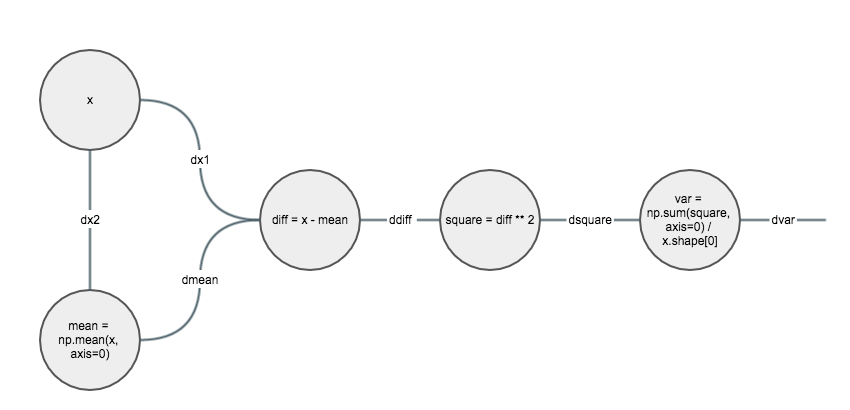# Backward 总结

### Computation graphdsquare = dvar / x.sahpe
ddiff = 2 * (x - mean) * dsquare
dmean = -np.sum(ddiff, axis=0)
dx1 = ddiff
dx2 = dmean / x.shape
dx = dx1 + dx2


Backpropagation, Intuitions